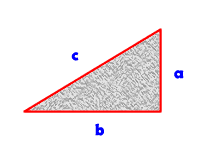if you know two sides of a triangle ,how do you figure side number three?

0  Views: 342 Answers: 1 Posted: 11 years ago

According to the Pythagorean Theorem, in a right triangle (a triangle of which one angel is of 90 degrees - a right angle)  the rule of the sentence a2 + b2 = c2 applies, when ’a' and 'b' represent the two sides next to the right angle.

If the lengths of the two sides are known, calculation according to a2 + b2 = c2 will give the length of c, which is the third line - the hypotenuse.### Top contributors in Homework Help category

ROMOS
Karma: 15225

country bumpkin
Karma: 10395

Benthere
Karma: 8625

Colleen